# Binary Splitting

## By Alexander Yee

(Last updated: February 18, 2019)

Back To:

y-cruncher can compute a variety of constants and simple functions. Most of these involve an infinite series of some sort. In order to efficiently sum up these series, it uses a technique called Binary Splitting.

As of version 0.7.7, y-cruncher has 4 different Binary Splitting implementations:

• Hyperdescent - A simple 2-variable recursion that handles series like the exp(x).
• CommonP2B3 - The standard 3-variable recursion that can handle most Hypergeometric Series.
• BinaryBBP - An optimized special case of CommonP2B3 for base 2 BBP-type formula.
• BrentMcMillanAB - A specialized implementation for the Brent-McMillan formula for the Euler-Mascheroni Constant.

In the past, there were separate implementations for every single constant and algorithm. But multiple refactorings and cleanups have consolidated most of them under the same generic implementation.

Hyperdescent - A Simple 2-Variable Recursion

This is a simple 2-variable Binary Splitting recursion that handles exp(1) and other similar series with a trivial numerator and a polynomial denominator.

Scope:

Mathematically, the Hyperdescent recursion in y-cruncher evaluates the following series: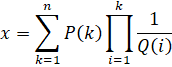where P(k), and Q(k) are arbitrary functions of k that return a real integer. The only restrictions are that they are easy to evaluate and the integer produced is reasonably small. In practice, both of them will be polynomials with word-sized integer coefficients. Furthermore, Q(k) will usually completely factorize.

This series type is really only useful for computing e. But it can also do other silly things like:

• exp(1/x) for integer x.
• sin(1/x), cos(1/x), and their hyperbolic variants for integer x.
• J0(1/x) and I0(1/x) for integer x where J() and I() are the Bessel and modified Bessel functions of the first kind.

In the past, this series was called, "eTaylor" in y-cruncher's source code since it was specialized for exp(1) and exp(-1). But starting from version 0.7.7, the implementation has been generalized and renamed to allow for other things that fit the series type.

The name, "Hyperdescent" describes the behavior of the series where each term "descends" very quickly. Thus, this series type converges very quickly.

The Recursion:

The Binary Splitting recursion for the series is as follows: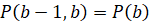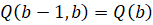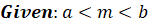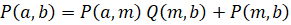Restrictions:

• Q(k) is non-zero for all integer k > 0. Otherwise a divide-by-zero will occur.

Convergence:

This series type is always convergent provided that Q(x) isn't 1 or -1.

Computational Speed:

When Q(x) is a constant, the series reduces to a geometric sequence which is linearly convergent.

When Q(x) is of degree 1 or higher, the series is superlinearly convergent. The rate of convergence increases for later terms.

All series of this type, regardless of P(x) and Q(x), asympotically have the same computational speed. As the # of digits approaches infinity, the ratio of the speeds between any two series of this type approaches 1. It also doesn't matter whether Q(x) is a constant or a polynomial.

The run-time complexity for this series type is O(N log(N)2) where N is the number of digits.

The only exception to the above is when Q(x) is a constant that's divisible by two. When that happens, y-cruncher's trailing zero optimizations will speed up the series by a constant factor thus preventing it from approaching the common limit.

CommonP2B3 - The Standard 3-Variable Recursion

This is the standard 3-variable Binary Splitting recursion that appears almost everywhere.

Scope:

Mathematically, the CommonP2B3 recursion in y-cruncher evaluates the following series: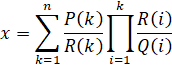where P(k), Q(k), and R(k) are arbitrary functions of k that return a real integer. The only restrictions are that they are easy to evaluate and the integer produced is reasonably small. In practice, all 3 of them will be polynomials with word-sized integer coefficients. Furthermore, Q(k), and R(k) will usually completely factorize.

This series type is very general and encompasses all hypergometric series of rationals. The vast majority of the popular constants can be computed with this type of series. y-cruncher doesn't actually use the name "Hypergeometric" because the CommonP2B3 series type is more general than that and "Hypergeometric function" has a very specific and well-defined meaning in math.

The name "CommonP2B3" is what is used in y-cruncher's source code. "B3" stands for the number of variables in the recursion. "P2" is the number of variables needed to obtain the final result.

As of y-cruncher v0.7.7, the CommonP2B3 recursion is used for all of the following series:

The Recursion:

The Binary Splitting recursion for the series is as follows: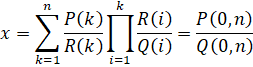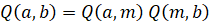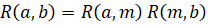Restrictions:

• Q(k) and R(k) are non-zero for all integer k > 0. Otherwise a divide-by-zero will occur.
• Limit k -> infinity of |R(k) / Q(k)| must be less than 1. This is needed to ensure linear or better convergence.
• |R(k) / Q(k)| must be less than 1 for all integer k > 0. Failing this means the series is not always converging. While this is mathematically fine since only the asymptotics matter, it can still wreck havoc on the implementation as it becomes difficult for y-cruncher to "predict" what it will do.

In practice, both Q(k) and R(k) will have no zeros for k >= 1. So both polynomials will be at their asymptotic behavior for the range that the series cares about.

Convergence:

If the goal is to sum up an infinite series, we must understand the convergence behavior.

Assuming P(k), Q(k), and R(k) are all polynomials with real coefficients, we can enumerate all the possibilities:

• If R(k) is of greater degree than Q(k), the series is divergent.
• If R(k) is of lower degree than Q(k), the series is superlinearly convergent.
• If R(k) and Q(k) are of the same degree, we need to look at the coefficient of the highest degree:
• If R(k) has the larger coefficient, the series is divergent.
• If Q(k) has the larger coefficient, the series is linearly convergent. This is the usual case. The ratio of the coefficients is the rate of convergence.
• If Q(k) and R(k) have the same magnitude coefficient, things get fuzzy as you enter the region of conditional and logarithmic convergence/divergence. For the purposes of high precision computation, none of these are acceptable so we won't go further into this.

All of the above assume that Q(k) and R(k) are non-zero for all integer k > 0.

• If R(k) is ever zero, the series terminates.
• If Q(k) is ever zero, it's a divide-by-zero.
• If both R(k) and Q(k) are both zero at the same time, the multiplicities will matter, but it's not as simple as it looks. Let's not go there.

y-cruncher will blow up on any of these cases even if they are mathematically sound.

Computational Speed:

Evaluating the computational speed of the series will depend on whether the series is linearly or superlinearly convergent. Superlinearly convergent series are less common and harder to analyze. So instead we'll focus on linearly convergent series.

Assuming P(k), Q(k), and R(k) are well-behaved polynomials with small and real integer coefficients, the relative asymptotic cost to summing up the series is given by: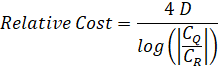Where:

• D is the degree of polynomials Q(k) and R(k). They will always be the same degree. If they aren't, then the series isn't linearly convergent.
• CR is the coeffcient of the highest order term of R(k).
• CQ is the coeffcient of the highest order term of Q(k).
• The factor of 4 is the weighting factor that's derived from the number of multiplications in the recursion. This allows series of different types with the same run-time complexity to be directly compared.

Things to note:

• Lower cost is better. The scale is linear. So if series A has twice the cost of series B, series A will actually take twice as long (asymptotically).
• log(CQ/CR) is the per-term convergence rate. If it is negative, the series is divergent.
• D is the cost of processing one term. If D is zero, the series reduces to a simple geometric sequence which is asympotically faster to compute.

Both the convergence rate and the polynomial degree are equally important. While many academic papers focus on finding fast converging series in terms of the number of bits or digits per term, they often do not weigh it against the cost of each individual term when estimating the actual speed of the series.

The run-time complexity for this series type is:

• O(N log(N)3) when the series is linearly convergent. (Q(k) same degree as R(k))
• O(N log(N)2) when the series is superlinearly convergent. (Q(k) higher degree than R(k))

where N is the number of digits.

Deriving Recursions:

You find an interesting formula and you don't know how to derive a Binary Splitting recusion for it. Here's a walk-through using Guillera's formula for Catalan's Constant.

Most of this is pretty self-explanatory, so we won't go into too much detail.

Original series: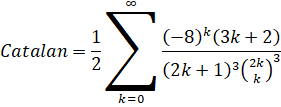Unpack the binomial into its factorials.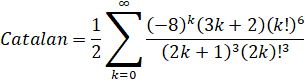Unpack the factorials and exponentials into products:Rearrange the formula to fit it into the CommonP2B3 series form.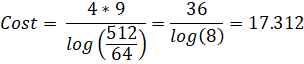And voila! We have a Binary Splitting recursion for Guillera's formula for Catalan's Constant with a cost of 17.312.

Optimizing Recursions:

Once you have a recursion, the next step is to optimize it. Recall the cost formula for linearly convergent series:Polynomial degree D is very important to the speed of the series. Therefore, there is a lot to gain by reducing it. One way to that is to remove common factors. Fundumentally, Binary Splitting is just a way of symbolically summing up a series of rationals. So it make sense to remove common factors between the numerators and denominators.

For the CommonP2B3 series, factors that are common to all 3 variables (P, Q, and R) can be removed. This applies to both constants as well as polynomial factors. Removal of polynomial factors will provide proportional speedups. Removal of constants will provide speedups for small computations where k doesn't get very large.

Here, we will discuss 3 ways to remove common factors:

1. Remove at the recursion end-points.
3. Remove globally.

Removal of Factors at End-Points:

Starting with the recursion we drived earlier for Guillera's formula for Catalan's Constant, we can see that the factor (2k)3 is common to all 3 polynomials.

Remove factors common to all 3 variables: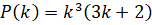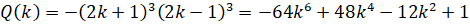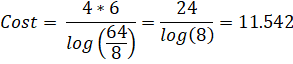This drops the degree from 9 to 6, thus removing 1/3 of the cost! This optimization should always done because it's easy to do and has no strings attached.

Removal of Factors across Adjacent Terms:

Sometimes, factors that cannot be removed at the end-points can be removed by combining adjacent terms. Let's do that to the example above: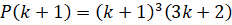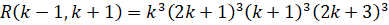Now we can see that the term (2k+1)3 is common to all 3 polynomials. Once we remove it and do an index substitution, we reach: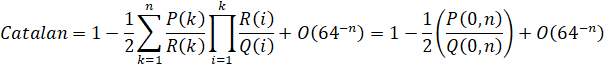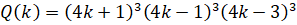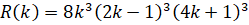The cost now drops to 8.656, which is another third faster from before. Note that since this can be applied to arbitrary pairs of adjacent terms, you effectively have an "infinite chain" of common factors that can be removed. So while you can merge an arbitrary number of adjacent terms, you will get increasingly complicated polynomials at the recursion end-points.

If such cases arise, it is worth examining the formula itself to see if anything can be optimized. If we can "shift" the index of Q so that it "lines up" with R...Observe that the (2k+1)3 term can be merged into the ((2k)!)3:Unpack the factorials: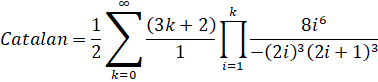Rearrange the formula to fit it into the CommonP2B3 series form.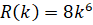Remove factors common to all 3 variables: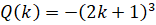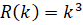Now we reach our final solution at a cost of 5.771. By merging away the (2k+1)3 term, it shifts the entire Q variable so that the common factors which were originally in adjacent terms, are now in the same term. This allows them to be eliminated by end-point factorization.

Adjacent term factorization is not always applicable. Catalan Guillera happens to be one of the few. But it does illustrate how slight modifications to a formula that's taken fresh out of a publication can lead to large differences in performance.

Generally speaking, it is not uncommon to have a series where it is possible to do adjacent term factorization, but it is not possible "shift" the series to remove all of them at once. Thus you end up in the unsatisfying situation of merging a finite number of terms while leaving some common factors behind.

Removal of Factors Globally:

In most cases, there are many more factors that cannot be eliminated by either of the methods above. This is because the common factors come from different values of k which are "far apart". Eliminating these factors is more complicated.

One of such approaches is to keep the prime-factorizations of Q(k) and R(k) along with their normal representations. Then at each stage of the recursion, compute the GCD from the prime factorization and remove it by division. This method is colloquially called, "GCD Factorization".

When applied to Pi Chudnovsky, speedups of 20 - 30% have been reported.

y-cruncher currently does not do GCD factorization for various reasons. It's been on the radar for years, but has been put off repeatedly.

Special Cases:

This last optimization doesn't have anything to do with removing factors. It's about removing entire recursion variables!

For certain inputs, the 3-variable recursion is unnecessary and it's possible to do away with one or more variables.

When R(k) = 1, the series reduces to the Hyperdescent series which is asympotically faster. exp(1) and exp(-1) both fall into this category.

For BBP-type formula in base 2, Q(k) becomes a power-of-two multiple of R(k). This power-of-two can be absorbed into the P(k) polynomial as the floating-point exponent, thereby eliminating the need for the Q(k) variable. This special case is handled in the next section.

Binary BBP - A 2-Variable Recursion for BBP-type Formula

As mentioned in the previous section, BBP-type formula can be evaluated faster than plugging it into the CommonP2B3 series. This "BinaryBBP" pattern is similar to the CommonP2B3 pattern, but with only 2 variables and the types of the variables have been relaxed from integers to scaled integers. (aka floating-point with no rounding)

Scope:

Mathematically, the BinaryBBP recursion in y-cruncher evaluates the following series: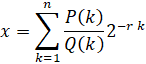where P(k) and Q(k) are arbitrary functions of k that return a real integer and r is a positive integer. Again, the only restrictions are that they are easy to evaluate and the integer produced is reasonably small. In practice, P(k) will be a constant and Q(k) will be a power of a 1st order polynomial with small coefficients.

As of y-cruncher v0.7.6, the only built-in constant/algorithm that uses this pattern is Catalan Huvent.

The Recursion:

The Binary Splitting recursion for the series is as follows: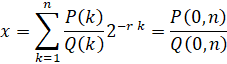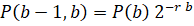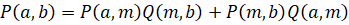All numbers will need to be represented as scaled integers with a base 2 exponent. So the addition in the P variable will be a shift-add.

Restrictions:

• Q(k) is non-zero for all integer k > 0. Otherwise a divide-by-zero will occur.
• r needs to be a positive real integer.

Not too many rules here since there is a lot less room for things to break.

Convergence:

Provided the restrictions above are followed, the BinaryBBP series is always linearly convergent.

Computational Speed:

Again assuming P(k), and Q(k) are well-behaved polynomials with small and real integer coefficients, the relative asymptotic cost to summing up the series is given by: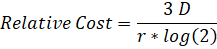Where:

• D is the degree of polynomial Q(k).
• The factor of 3 is the weighting factor that's derived from the number of multiplications in the recursion.

Things to note:

• Lower cost is better. The scale is linear. So if series A has twice the cost of series B, series A will actually take twice as long (asymptotically).
• r * log(2) is the per-term convergence rate. If it's negative, the series is divergent.
• D is the cost of processing one term. If D is zero, the series reduces to a simple geometric sequence which is asympotically faster to compute.

The run-time complexity for this series type is:

• O(N log(N)3) when Q(k) is of degree 1 or higher.
• O(N log(N)2) when Q(k) is of degree 0.

where N is the number of digits.

Optimizations:

Since the BinaryBBP series is simpler than the CommonP2B3 series, there's a lot less room for optimizations. Removal of common factors applies to the BinaryBBP series just as it does with the CommonP2B3. But in practice, P(k) will be a constant. So there really isn't any thing that can be optimized.